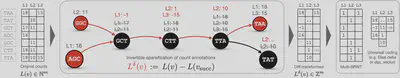# Lossless Indexing with Counting de Bruijn Graphs

### Abstract

Sequencing data is rapidly accumulating in public repositories. Making this resource accessible for interactive analysis at scale requires efficient approaches for its storage and indexing. There have recently been remarkable advances in building compressed representations of annotated (or colored) de Bruijn graphs for efficiently indexing k-mer sets. However, approaches for representing quantitative attributes such as gene expression or genome positions in a general manner have remained underexplored. In this work, we propose Counting de Bruijn graphs (Counting DBGs), a notion generalizing annotated de Bruijn graphs by supplementing each node-label relation with one or many attributes (e.g., a k-mer count or its positions). Counting DBGs index k-mer abundances from 2,652 human RNA-Seq samples in over 8-fold smaller representations compared to state-of-the-art bioinformatics tools and yet faster to construct and query. Furthermore, Counting DBGs with positional annotations losslessly represent entire reads in indexes on average 27% smaller than the input compressed with gzip for human Illumina RNA-Seq and 57% smaller for PacBio HiFi sequencing of viral samples. A complete searchable index of all viral PacBio SMRT reads from NCBI’s SRA (152,884 samples, 875 Gbp) comprises only 178 GB. Finally, on the full RefSeq collection, we generate a lossless and fully queryable index that is 4.4-fold smaller than the MegaBLAST index. The techniques proposed in this work naturally complement existing methods and tools employing de Bruijn graphs and significantly broaden their applicability: from indexing k-mer counts and genome positions to implementing novel sequence alignment algorithms on top of highly compressed graph-based sequence indexes.

Type
Publication
In RECOMB 2022Fig. 3: Extraction of k-mer coordinates from sequence $\texttt{ACTAGCTAGCTAG}$ for $k=3$ (panel A) and subsequent compression with a diff-transform (panel B), where the coordinates at a node's successor are expected to be the same but incremented by +1, as these nodes are likely to be consecutive in the input sequence(s). The symmetric set difference $A\Delta B := (A\cup B)\setminus(A\cap B)$ is used as a diff-operation. The inverse transform is performed losslessly by $L(v)=(L(v_\text{succ})\Delta L^\delta(v))\ominus1$, which follows from the following property: $(A\Delta B)\Delta B = A$ $\forall A,B$.Fig. 2: A schematic diagram illustrating encoding of k-mer counts in m columns with the proposed approach. Circles represent nodes of a de Bruijn graph. Edges are shown as arrows. Red nodes represent anchor nodes and red edges represent paths to the diff successors. The transformed counts are shown in red (e.g., compare L1 : −1 for k-mer $\texttt{GCT}$ after the transform and L1 : 17, L2 : 11 before). Then, the diff-transformed matrix is decomposed into an indicator binary matrix stored in the compressed Multi-BRWT representation supporting the rank operation on the columns and arrays storing non-zero diffs.Fig. 1: The proposed representation of sparse matrices in compressed form. Panel A: General scheme for sparse matrices with abstract attributes, where the non-assigned attributes are eliminated by an indicator binary matrix stored in a compressed representation (e.g., Multi-BRWT) supporting the rank operation on its columns to enable the access to the corresponding attribute for any given cell of the matrix. These attributes are stored separately, typically in a form of compressed arrays. Panel B: The scheme applied to a single column with integer values (e.g., k-mer counts) and the query algorithm (e.g., the count of k-mer $i$ is retrieved as $A_1[\text{rank}(B_1,i)]$. Empty cells in grey represent zeros. Panel C: The scheme applied to a single column where each cell is a set of numbers, or a tuple (e.g., representing k-mer coordinates). The ”zero” attributes (empty sets) are eliminated with an indicator bitmap and the non-empty sets are encoded in an array that holds all numbers and a delimiting bitmap.##### Mikhail Karasikov
###### PhD Student

My research interests include machine learning and bioinformatics.